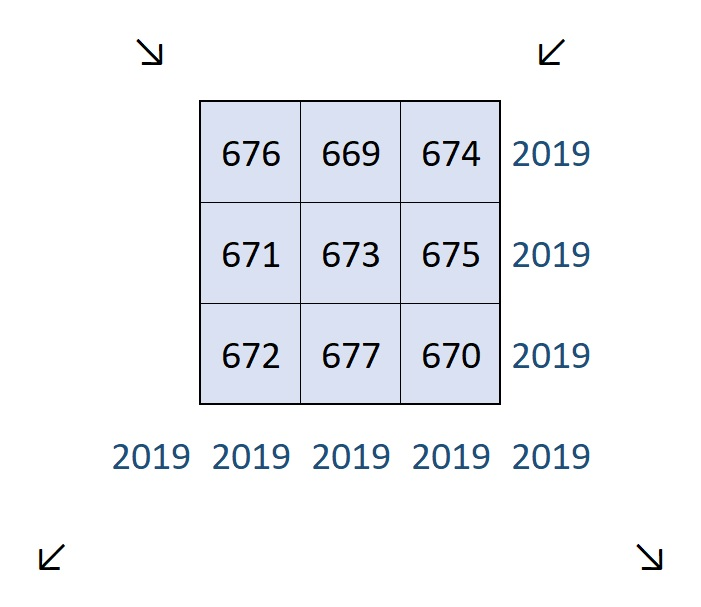# (1346÷2)×3 = 2019 Magic or Square?

I was inspired to make a 3 × 3 Magic Square where every number is different but the numbers in each row, column and diagonal added together equal the same number, 54:I made it by taking a regular 3 × 3 Magic Square and adding 13 to each of its numbers.

What inspired me to do that? This magical tweet of a palindromic Magic Square for the Year 2019:

Yeah, I know my magic square isn’t quite as impressive. It might be more square than it is magic. It’s also less impressive than this number 2019 spelled out using fifty-one
4 × 4 Magic Squares.

Maybe you will be more impressed by this magic square that has 2019 as its Magic Sum?I could make that magic sum because 2019 is divisible by 3. Why is 673 in the center? Because 2019÷3 = 673.

673 × 2 = 1346. I’m sharing these magic squares in this post I’ve numbered 1346. In case you haven’t figured it out (1346÷2)×3 = 2019. Happy New Year!

Here’s more about the number 1346:

• 1346 is a composite number.
• Prime factorization: 1346 = 2 × 673
• 1346 has no exponents greater than 1 in its prime factorization, so √1346 cannot be simplified.
• The exponents in the prime factorization are 1, and 1. Adding one to each exponent and multiplying we get (1 + 1)(1 + 1) = 2 × 2 = 4. Therefore 1346 has exactly 4 factors.
• The factors of 1346 are outlined with their factor pair partners in the graphic below.1346 is the hypotenuse of a Pythagorean triple:
770-1104-1346 which is 2 times 385-552-673

This site uses Akismet to reduce spam. Learn how your comment data is processed.